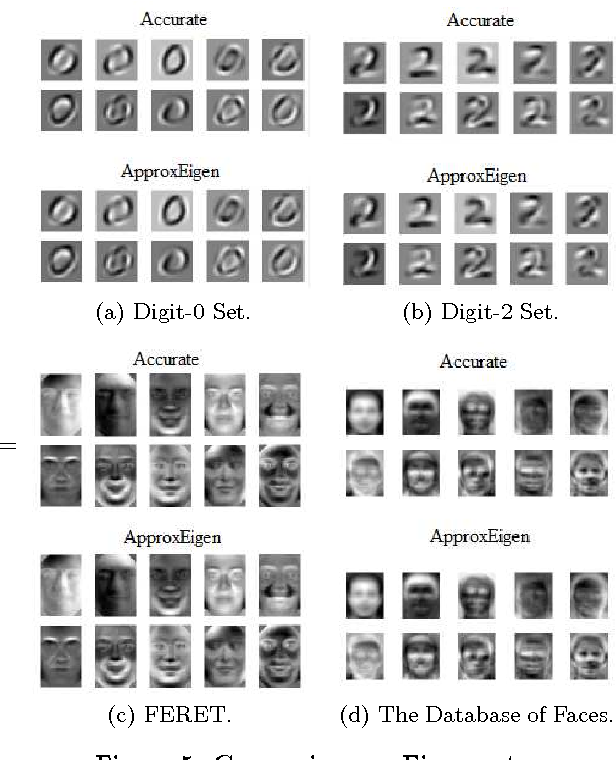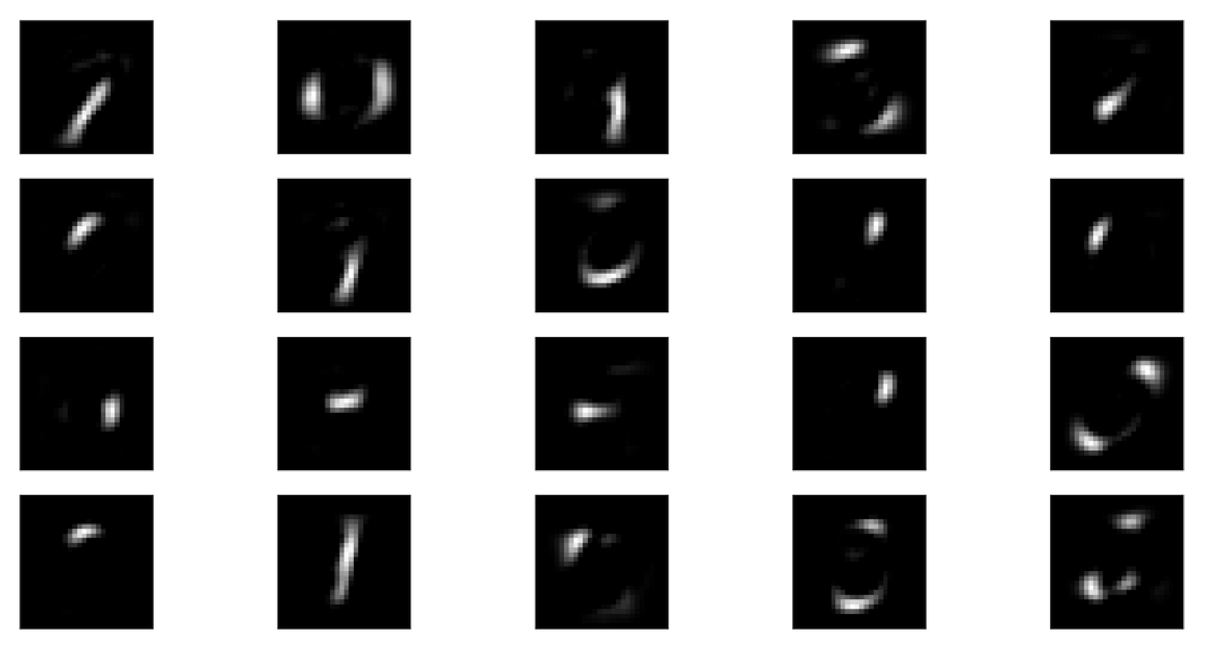4 Matching Annotations
1. Mar 2019
2. iphysresearch.github.io iphysresearch.github.io
1. O-GAN: Extremely Concise Approach for Auto-Encoding Generative Adversarial Networks

URL

3. Feb 2019
4. iphysresearch.github.io iphysresearch.github.io
1. Unsupervised speech representation learning using WaveNet autoencoders

我们通过将自动编码神经网络应用于语音波形来考虑无监督提取有意义的语音潜在表示的任务。目标是学习能够从信号中捕获高级语义内容的表示，例如，音素身份，同时不会混淆信号中的低级细节，例如底层音高轮廓或背景噪音。自动编码器模型的行为取决于应用于潜在表示的约束类型。我们比较了三种变体：简单的降维瓶颈，高斯变分自动编码器（VAE）和离散矢量量化VAE（VQ-VAE）。我们根据说话人的独立性，预测语音内容的能力以及精确重建单个谱图帧的能力来分析学习表征的质量。此外，对于使用VQ-VAE提取的差异编码，我们测量将它们映射到电话的容易程度。我们引入了一种正则化方案，该方案强制表示集中于话语的语音内容，并报告性能与ZeroSpeech 2017无监督声学单元发现任务中的顶级条目相当。 【translated by 谷歌翻译】

【摘要自机器之心】：

论文《Unsupervised speech representation learning using WaveNet autoencoders》介绍了通过将自编码神经网络用到语音波形提取语音中有意义的隐藏表征的无监督任务。目的是学习到一种能够捕捉信号中高层次语义内容的表征，同时又能够对有背景噪声或者潜在基频曲线（underlying pitch contour）的信号中的扰乱信息足够稳定。自编码器模型的行为由应用到隐藏表征的约束所决定。在此论文中，作者对比了三种变体：简单降维瓶颈、高斯变分自编码器和离散向量量化VAE。而后，作者对预测语音内容的能力等进行了分析。

URL

5. Jan 2019
6. iphysresearch.github.io iphysresearch.github.io
1. Understanding Geometry of Encoder-Decoder CNNs

由于计算机视觉，医学成像等各种逆问题的优异性能，使用卷积神经网络（CNN）架构的编码器 - 解码器网络已被广泛用于深度学习文献中。然而，仍然难以获得相干几何学视图为何如此架构提供了理想的性能。最近对神经网络的普遍性，表现力和优化景观的理论认识以及卷积框架理论的启发，在这里我们提供了一个统一的理论框架，有助于更好地理解编码器 - 解码器CNN的几何。我们的数学框架表明，编码器 - 解码器CNN架构与使用组合卷积帧的非线性基表示密切相关，其可表达性随着网络深度呈指数增长。我们还展示了跳过连接的可表达性和优化环境的重要性。

URL

7. Oct 2018
8. 192.168.199.102:5000 192.168.199.102:5000
1. 从 components-PCA 到 Autoencoder

根据之前通过 SVD 矩阵分解得到的结论： u = w

和公式：$$\hat{x} = \sum_{k=1}^Kc_kw^k \approx x-\bar{x}$$

再结合线性代数的知识，我们可以得到，能让 reconstruction error 最小的 c 就是：

$$c^k = (x-\bar{x})\cdot w^k$$

结合这两个公式，我们就可以找到一个 Autoencoder 结构：

1. $$(x-\bar{x})\cdot w^k = c^k$$
2. $$\sum_{k=1}^Kc^kw^k = \hat{x}$$

$$\begin{vmatrix} x_1 - \bar{x} \\ x_2 - \bar{x} \\ .\\.\\. \end{vmatrix} \Rightarrow \begin{vmatrix} c^1 \\ c^2 \end{vmatrix} \Rightarrow \begin{vmatrix} \bar{x_1} \\ \bar{x_2} \\ .\\.\\. \end{vmatrix}$$

autoencoder 的缺点 --- 无法像 PCA 一样得到完美的数学解

这样一个线性的 autoencoder 可以通过 Gradient Descent 来求解，但是 autoencoder 得到的解只能无限接近 PCA 通过 SVD 或者 拉格朗日乘数法的解，但不可能完全一致，因为 PCA 得到的 W 矩阵是一个列和列之间都相互垂直的矩阵，autoencoder 确实可以得到一个解，但无法保证参数矩阵 W 的列之间相互垂直

autoencoder 的优点 --- 可以 deep，形成非线性函数，面对复杂问题更 power

PCA 只能做压扁不能做拉直

就像下面显示的这样，PCA 处理这类 manifold（卷曲面）的数据是无能为力的。他只能把数据都往某个方向压在一起。而 deep autoencoder 可以处理这类复杂的降维问题：how many the principle components?

你要把数据降到几维度，是你可以自己决定的，但是有没有什么比较好的数学依据来hint这件事情呢？

常用的方法是：

$$eigen\ ratio\ = \frac{\lambda_i}{\lambda_1 + \lambda_2 + \lambda_3 + \lambda_4 + \lambda_5 + \lambda_6}$$

• $$\lambda$$ ： eigen value of Cov(x) matrix

我们求解 PCA 的函数的时候给出的结论是：

W 的列是 $$S=Cov(x)$$ 的topmost K 个 eignen values 对应的 eigen vectors.

eigen values 的物理意义是降维后的 z 空间中的数据集在这一维度的 variance。

在决定 z 空间的维度之前，我们引入一个指标： eigen ratio，这个指标有什么用呢？他能帮助我们估算出前多少个 eigen vector 是比较合适的。

假设我们预先希望降维到 6 dimension，那么我们就可以通过之前学到的方法得到 6 个 eigen vector 和 6 个 eigen value, 同时也可以得到 6 个 eigen ratio.

通过这 6 个 eigen ratio 我们就可以看出谁提供的 variance 是非常小的（而我们的目标是找到最大 Variance(z)）。eigen ration 太小表示映射之后的那个维度，所有的点都挤在一起了，他没什么区别度，也就是提供不了太多有用的信息。

之前没有提过，component 的维度应该是与原始 x 样本的维度是一致的，因为你可以把 component 看成是原始维度的一种组合（笔画 <- 像素）。

以宝可梦为例，说明： 如果把原本 6 个维度的宝可梦，降维到4维度，可以发现的是这个 4 个 componets 大概的物理意义是：

1. 1st_component: 强度，对应的原始样本 6 维度都是正系数。
2. 2nd_component: 防御力(牺牲速度)，对应的原始样本中 'Def' 最高，'Speed' 最低（负值）
3. 3rd_component: 特殊防御（牺牲攻击和生命值），对应的原始样本中 'Sp Def' 最高，'HP','Atk'最低（都是负值）
4. 4th_component: 生命值（牺牲攻击力和防御力），对应的原始样本中的'HP'最高， 'Atk' 'Def' 最低（都是负值）

从实际应用看 PCA 得到的 'component'

'component' 不一定是 ‘部分’。

• 对手写数字图片进行降维

• 对人脸图片进行降维上面的图片所展示的似乎异于我们期望看到的啊！

我们一直强调 component 似乎是一种部分与整体的感觉，而这里给出的图片似乎是一种图层的感觉 --- 每一张 'component' 给出的不是笔画和五官，而是完整的数字和脸的不同颜色or阴影。

进一步分析 PCA 得到的 'component' --- 滤镜

提出一种可能性：先看之前的公式，

$$img_9 = c_1w_1 + c_2w_2 + ...$$

由于我们并没有限制，factor 系数的符号（factor 可正可负），所以他极有可能做的一件事情是，

为了生成图片 数字9，我的第一个component可以比较复杂，比如图片数字8，给与其正的factor，第二个component可以是一个0,给与其正的factor，第三个component可以是一个 6，给与其负值的factor

这样 img8 + img0 - img6 看上去就约等于 img9 了。

如何能使得 component 就是‘部分’

PCA 我们使用的分解是 SVD 或者 lagrange multiplier. 这种方法无法保证我得到的 component 和 其对应的 factor 都是正的。于是可以使用 NMF(non-negative matrix factorization)

NMF:

1. forcing all factors be non-negative
2. forcing all components be non-negative

李宏毅老师没有具体讲解这个方法，只列出索引：

Algorithms for non-negative matrix factorization

如下图, NMF 使用的 components 更像是 ‘部分’ 而不是 ‘滤镜’